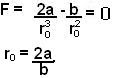Friday, May 2, 2008

Irodov Problem 1.131

Force is the negative gradient of potential i.e.. In spherical coordinates this is given bySince in this problem, U is only a function or r, i.e. depends only on the distance from the center, the force acting on it must be radial (since all other partial derivatives will be zero in the above expression). Thus, the force acting radially outwards (away from the center) is given by,At the equilibrium point the net force will be zero, this meansThe concept of stability has been explained in the solution to problem 1.109. The idea being that under small perturbations the body must have a tendency to return to its original equilibrium point. Consider a small perturbationabout the equilibrium point. The force acting on the body will be,The easiest way to check stability is to linearize the above expression for small perturbation about the equilibrium point and see if the force is restoring. So using Taylor's expansion and ignoring all terms with power higher than 1 we have,The force thus always acts in the opposite direction of the perturbation (as seen by the negative sign), this means that the force acts in a manner so as to restore the particle to its equilibrium position. In fact for small perturbations the motion is simple harmonic. In other words the equilibrium point is stable.

b)At the extrema the first derivative should be zero so at the point where magnitude if attractive force is maximum, so we have,The value of this force can be obtained by simply substituting in the expression for force and is given by,